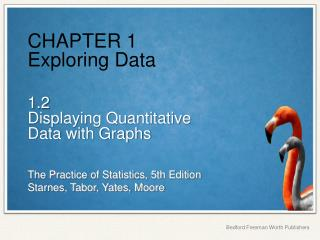DownloadDownload PresentationCHAPTER 1 Exploring Data

# CHAPTER 1 Exploring Data

Télécharger la présentation## CHAPTER 1 Exploring Data

- - - - - - - - - - - - - - - - - - - - - - - - - - - E N D - - - - - - - - - - - - - - - - - - - - - - - - - - -
##### Presentation Transcript

1. CHAPTER 1Exploring Data 1.2Displaying Quantitative Data with Graphs

2. Displaying Quantitative Data with Graphs • MAKE and INTERPRET dotplots and stemplots of quantitative data • DESCRIBE the overall pattern of a distribution and IDENTIFY any outliers • IDENTIFY the shape of a distribution • MAKE and INTERPRET histograms of quantitative data • COMPARE distributions of quantitative data

3. Dotplots One of the simplest graphs to construct and interpret is a dotplot. Each data value is shown as a dot above its location on a number line. • How to make a dotplot: • Draw a horizontal axis (a number line) and label it with the variable name. • Scale the axis from the minimum to the maximum value. • Mark a dot above the location on the horizontal axis corresponding to each data value.

4. Examining the Distribution of a Quantitative Variable The purpose of a graph is to help us understand the data. After you make a graph, always ask, “What do I see?” • How to Examine the Distribution of a Quantitative Variable • In any graph, look for the overall pattern and for striking departures from that pattern. • Describe the overall pattern of a distribution by its: • Shape • Center • Spread • Note individual values that fall outside the overall pattern. These departures are called outliers. Don’t forget your SOCS!

5. Describing Shape When you describe a distribution’s shape, concentrate on the main features. Look for rough symmetry or clear skewness. A distribution is roughly symmetricif the right and left sides of the graph are approximately mirror images of each other. A distribution is skewed to the right (right-skewed) if the right side of the graph (containing the half of the observations with larger values) is much longer than the left side. It is skewed to the left (left-skewed) if the left side of the graph is much longer than the right side. Symmetric Skewed-left Skewed-right

6. Comparing Distributions Some of the most interesting statistics questions involve comparing two or more groups. Always discuss shape, center, spread, and possible outliers whenever you compare distributions of a quantitative variable. Compare the distributions of household size for these two countries. Don’t forget your SOCS!

7. Stemplots Another simple graphical display for small data sets is a stemplot. (Also called a stem-and-leaf plot.) Stemplots give us a quick picture of the distribution while including the actual numerical values. • How to make a stemplot: • Separate each observation into a stem (all but the final digit) and a leaf (the final digit). • Write all possible stems from the smallest to the largest in a vertical column and draw a vertical line to the right of the column. • Write each leaf in the row to the right of its stem. • Arrange the leaves in increasing order out from the stem. • Provide a key that explains in context what the stems and leaves represent.

8. Stemplots These data represent the responses of 20 female AP Statistics students to the question, “How many pairs of shoes do you have?” Construct a stemplot. Key: 4|9 represents a female student who reported having 49 pairs of shoes. 1 93335 2 664233 3 1840 4 9 5 0701 1 33359 2 233466 3 0148 4 9 5 0017 1 2 3 4 5 Stems Add leaves Order leaves Add a key

9. Stemplots When data values are “bunched up”, we can get a better picture of the distribution by splitting stems. Two distributions of the same quantitative variable can be compared using a back-to-back stemplot with common stems. Females Males Females 333 95 4332 66 410 8 9 100 7 Males 0 4 0 555677778 1 0000124 1 2 2 2 3 3 58 4 4 5 5 0 0 1 1 2 2 3 3 4 4 5 5 “split stems” Key: 4|9 represents a student who reported having 49 pairs of shoes.

10. Histograms Quantitative variables often take many values. A graph of the distribution may be clearer if nearby values are grouped together. The most common graph of the distribution of one quantitative variable is a histogram. • How to make a histogram: • Divide the range of data into classes of equal width. • Find the count (frequency) or percent (relative frequency) of individuals in each class. • Label and scale your axes and draw the histogram. The height of the bar equals its frequency. Adjacent bars should touch, unless a class contains no individuals.

11. Histograms This table presents data on the percent of residents from each state who were born outside of the U.S. Number of States Percent of foreign-born residents

12. Using Histograms Wisely Here are several cautions based on common mistakes students make when using histograms. • Cautions! • Don’t confuse histograms and bar graphs. • Don’t use counts (in a frequency table) or percents (in a relative frequency table) as data. • Use percents instead of counts on the vertical axis when comparing distributions with different numbers of observations. • Just because a graph looks nice, it’s not necessarily a meaningful display of data.

13. Data Analysis: Making Sense of Data • MAKE and INTERPRET dotplots and stemplots of quantitative data • DESCRIBE the overall pattern of a distribution • IDENTIFY the shape of a distribution • MAKE and INTERPRET histograms of quantitative data • COMPARE distributions of quantitative data